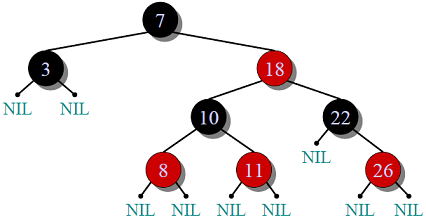Binary Search Tree (BST)

A binary search tree is a node-based binary tree data structure where each node has a comparable key (and associated value ) and satisfies the restriction that the key in any node is larger than the key in all nodes in that node’s left  sub-tree and smaller than the key in all nodes in that node’s right sub-tree. Each non-leaf node has exactly two nodes. Each child must either a leaf node or the root of another binary tree. BST’s are also dynamic data structure and the size of a BST is only limited by the amount of free memory in the operating system.

The main advantages of binary search tree is that it remains ordered, which provide quicker search times  than many other data structure.Binary Search Tree : A binary search tree of size 9 and depth 3, with root 8 and leaves 1, 4, 7 and 13

Properties of BST are following as :

1. One node is designed the root of the tree.
2. Each internal node  contains a key and has two sub-trees.
3. Each sub-tree is itself a binary search tree.
4. The leaves of the tree contain no key. Leaves are commonly represented by leaf or nil symbol, a Null pointer etc.
5. The left sub-tree of a node contains only nodes with keys strictly less than node’s key.
6. The right sub-tree of a node contains only nodes with keys strictly greater than the node’s key.

Time Complexity of BST in big O notation :

Space Search Insert Average Worst case O(n) O(n) O(log n) O(n) O(log n) O(n) O(log n) O(n)

Red & Black Tree

A Red-Black tree is a binary search tree with an extra bit of data per node, its colour, which can be either red or black. The extra bit of storage ensure an approximately balance tree by constraining how nodes are coloured from the root to the leaf. Thus, it is a data structure which is type of self-balancing binary search tree.

Red-Black Tree is a self-balancing Binary Search Tree (BST) where every node has following rules.

1. Every node has a colour either red or black.
2. Root of tree is always black.
3. There are no two adjacent red nodes (A red node cannot have a red parent or red child).
4. Every path same number of black nodes.Why Red-Black Trees?
Most of the BST operations (e.g search, max, min, insert, delete etc) take O(h) time where h is the height of the BST. The cost of these operations may become O(n) for a skewed Binary tree. If we make sure that height of the tree remains O(Logn) after every insertion and deletion, then we can guarantee an upper bound of O(Logn) for all these operations. The height of a Red Black tree is always O(Logn) where n is the number of nodes in the tree.

Comparison with AVL tree
The AVL trees are more balanced compared to Red Black Trees, but they may cause more rotations during insertion and deletion. So if your application involves many frequent insertions and deletions, then Red Black trees should be preferred. And if the insertions and deletions are less frequent and search is more frequent operation, then AVL tree should be preferred over Red Black Tree.